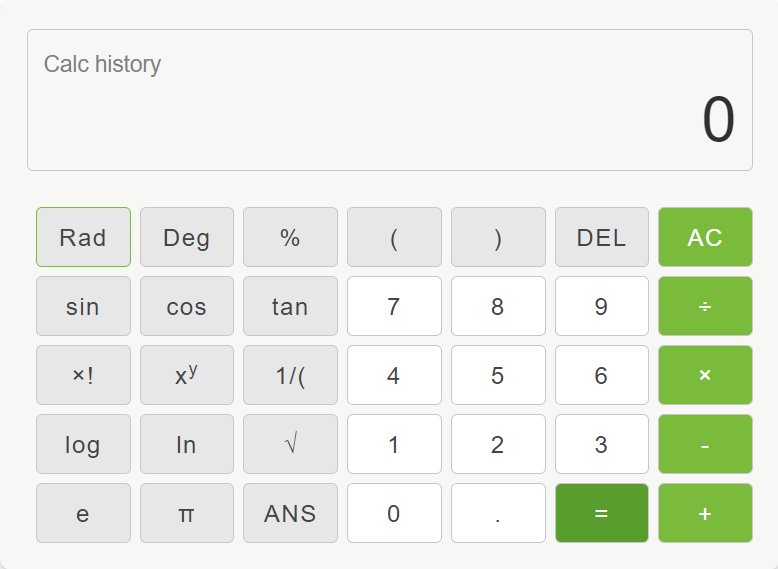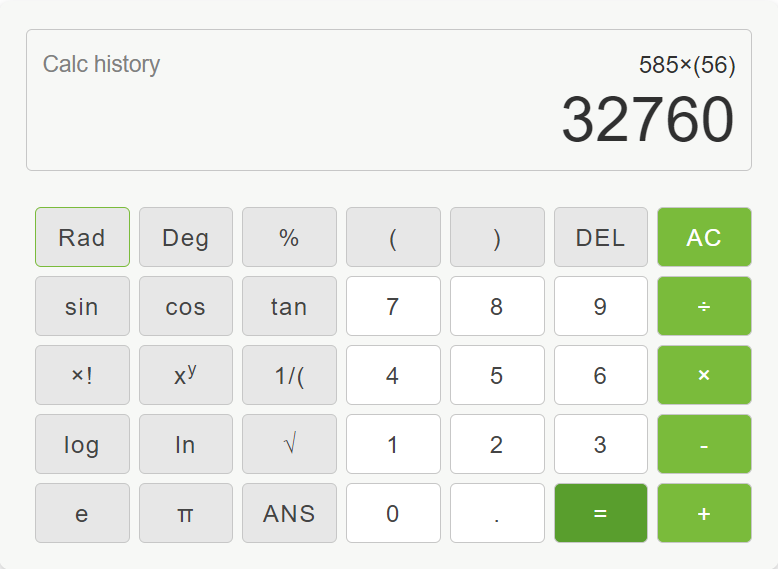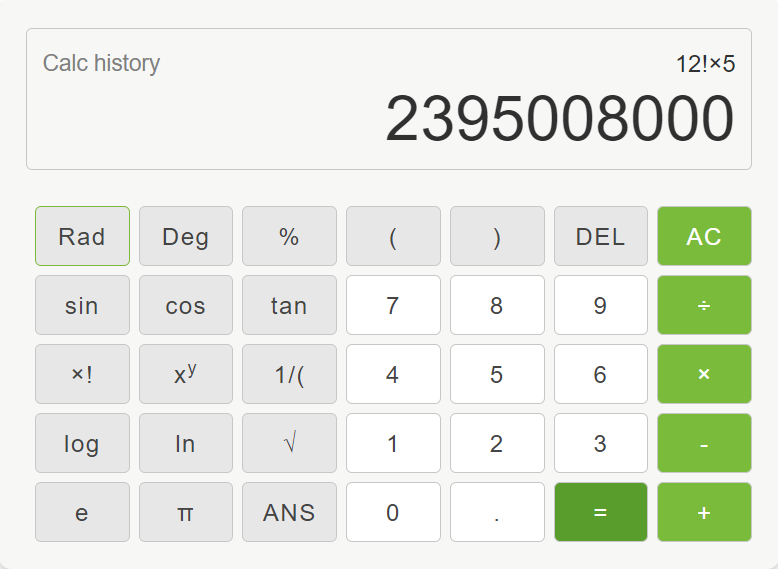# Calculator

The Mojeek calculator provides both simple and advanced mathematical functions in an easy-to-use way. Functions include basic calculations such as addition, subtraction, multiplication and division; whilst also allowing for scientific operations such as trigonometric, logarithmic and exponential functions.

In order to use the calculator, you can search using a query composed of a mathematical expression using the supported symbols listed below. Alternatively, you can enter “calculator” in the search bar to show the calculator function.

To operate, use the on-screen buttons, or click into the display field and enter an expression using your keyboard.This can be similarly triggered using the search barThe query you type in will then be displayed on the calculator, as if you had typed the same equation into the calculator.

## Functions, operators, and constants

Mojeek supports the following functions, operators, and constants:

Functions Operators
cos ^
cosh x *
log (base 10) ÷ /
lg (log base 2) +
ln %
sin -
sinh !
sqrt
tan
tanh
Constants
pi
e

Below are some examples of how the calculator feature can be used and some of the functions available.